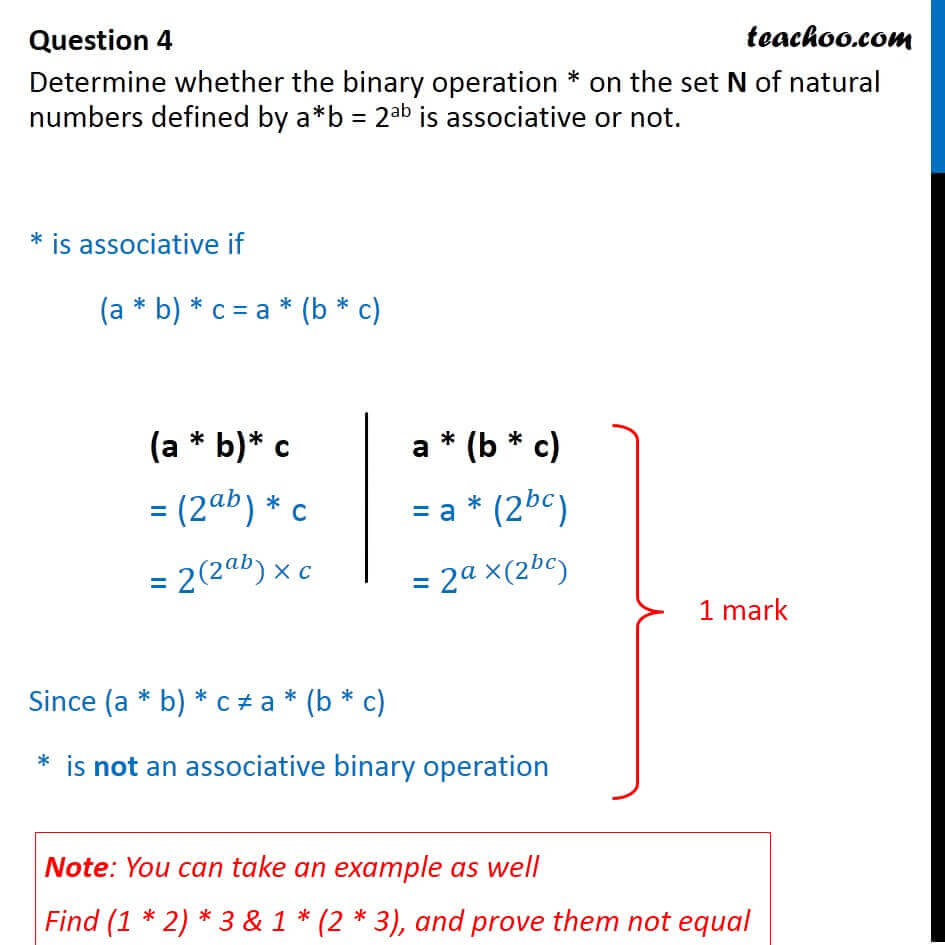CBSE Class 12 Sample Paper for 2018 Boards

Class 12
Solutions of Sample Papers and Past Year Papers - for Class 12 Boards

### Determine whether the binary operation * on the set N of natural numbers defined by a*b = 2 ab is associative or not.

This is a question of CBSE Sample Paper - Class 12 - 2017/18.Learn in your speed, with individual attention - Teachoo Maths 1-on-1 Class

### Transcript

Question 4 Determine whether the binary operation * on the set N of natural numbers defined by a*b = 2ab is associative or not. * is associative if (a * b) * c = a * (b * c) Since (a * b) * c a * (b * c) * is not an associative binary operation (a * b)* c = (2^ ) * c = 2^( (2 ^ ) ) a * (b * c) = a * (2^ ) = 2^( (2^ )) Note: You can take an example as well Find (1 * 2) * 3 & 1 * (2 * 3), and prove them not equal